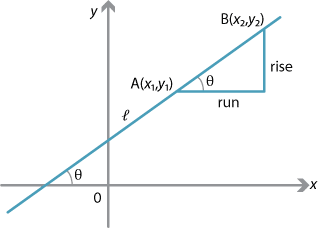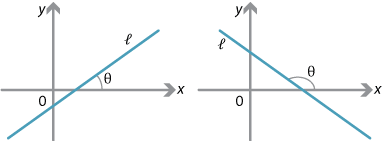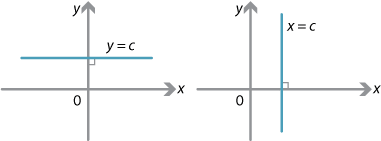## Content

### Gradients and the angle of inclination

Suppose $$l$$ is a line in the number plane not parallel to the $$y$$-axis or the $$x$$-axis.Detailed description

Let $$\theta$$ be the angle between $$l$$ and the positive $$x$$-axis, where $$0^\circ<\theta<90^\circ$$ or $$90^\circ<\theta<180^\circ$$. Suppose $$A(x_1,y_1)$$ and $$B(x_2,y_2)$$ are two points on $$l$$. Then, by definition, the gradient of the interval $$AB$$ is

$m = \dfrac{\text{rise}}{\text{run}} = \dfrac{y_2-y_1}{x_2-x_1}.$

From the diagram above, this is equal to $$\tan\theta$$. So $$\tan\theta$$ is the gradient of the interval $$AB$$. Thus the gradient of any interval on the line is constant. Thus we may sensibly define the gradient of $$l$$ to be $$\tan\theta$$.Detailed description

In the case where the line is parallel to the $$x$$-axis, we say that the gradient is 0. In the case where the line is parallel to the $$y$$-axis, we say that $$\theta=90^\circ$$.$$\theta$$ not defined no gradient $$\theta=90^\circ$$
Detailed description

#### Example

Find the gradients of the lines joining the following pairs of points. Also find the angle $$\theta$$ between each line and the positive $$x$$-axis.

1. $$(2,3)$$, $$(5,3)$$
2. $$(1,2)$$, $$(7,8)$$
3. $$(1,2)$$, $$(7,-4)$$
4. $$(2,3)$$, $$(2,7)$$

#### Solution

1. The line through $$(2,3)$$ and $$(5,3)$$ is parallel to the $$x$$-axis and so the gradient is 0.
2. The gradient is $$\dfrac{8-2}{7-1} = 1$$ and $$\theta = 45^\circ$$.
3. The gradient is $$\dfrac{-4-2}{7-1} = -1$$ and $$\theta =135^\circ$$.
4. The line is parallel to the $$y$$-axis, so the line has no gradient and $$\theta=90^\circ$$.

Exercise 5

Find the gradients of the lines joining the following pairs of points:

1. $$\Bigl(cp,\dfrac{c}{p}\Bigr)$$, $$\Bigl(cq,\dfrac{c}{q}\Bigr)$$
2. $$(ap^2,2ap)$$, $$(aq^2,2aq)$$
3. $$(a\cos\theta, b\sin\theta)$$, $$(a\cos\phi,b\sin\phi)$$.

Next page - Content - Intercepts and equations of lines